Courses

# Precipitation and General Aspects of Hydrology Civil Engineering (CE) Notes | EduRev

## Civil Engineering (CE) : Precipitation and General Aspects of Hydrology Civil Engineering (CE) Notes | EduRev

The document Precipitation and General Aspects of Hydrology Civil Engineering (CE) Notes | EduRev is a part of the Civil Engineering (CE) Course Water Resources Engineering.
All you need of Civil Engineering (CE) at this link: Civil Engineering (CE)

Question 1. A catchment may be idealised as a rectangle. There are three rain gauges located inside the catchment at arbitrary locations. The average precipitation over the catchment is estimated by two methods:     [2019 : 1 Mark, Set-I]
(i)    Arithmetic mean (PA), and
(ii)   Thiessen polygon (PT). Which one of the following statements is correct?
(a)    PA is always smaller than PT
(b)    PA is always equal to PT
(c)    There is no definite relationship between PA and PT
(d)    PA is always greater than P
Answer:
(c)
Solution:
The result from Thiessen polygon method is more accurate than arithmetic mean method. But there is no any close relationship between values obtained by Thiessen polygon method and Arithmetic mean method.
∴ There is no any relation between PA and PT.

Question 2. Rainfall depth over a watershed is monitored through six number of well distributed rain gauges. Gauged data are given below: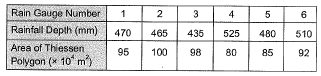The Thiessen mean value (in mm, up to one decimal place) of the rainfall is______      [2018 : 2 Marks, Set-I]
Solution:
Thiessen mean value of rainfall;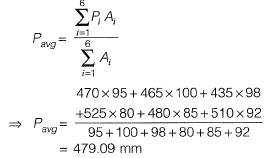Question 3. A catchment is idealized as a 25 km x 25 km square. It has five rain gauges, one at each corner and one at the center, as shown in the figure.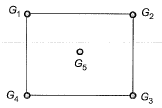During a month, the precipitation at these gauges is measured as G1 = 300 mm, G2 = 285 mm, G3 = 272 mm, G4 = 290 mm and G5 = 288 mm. The average precipitation (in mm, up to one decimal place) over the catchment during this month by using the Thiessen polygon method is      [2017 : 2 Marks, Set-II]

Solution: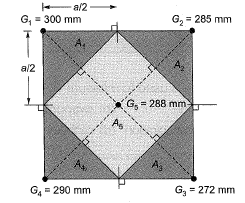Let sides of square be, a = 25 km Now area of the polygon is calculated as,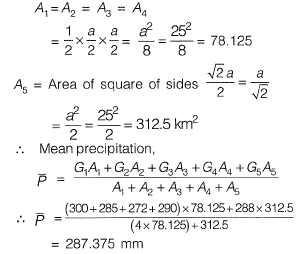Question 4. In a catchment, there are four rain-gauge stations, P, Q, Ft and S. Normal annual precipitation values at these stations are 780 mm, 850 mm, 920 mm and 980 mm, respectively. In the year 2013, stations Q, R and S, were operative but P was not. Using the normal ratio method, the precipitation at station P for the year 2013 has been estimated as 860 mm. If the observed precipitation at stations O and P for the year 2013 were 930 mm and 1010 mm, respectively; what was the observed precipitation (in mm) at station S for that year?      [2015 : 2 Marks, Set-I]
Solution:
Let Pp = Precipitation in 2013 at station P
Np = Normal annual precipitation at station P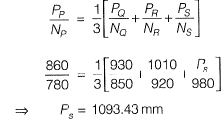Question 5. An isohyet is a line joining points of     [2013 : 1 Mark]
(a) equal temperature
(b) equal humidity
(c) equal rainfall depth
(d) equal evaporation
Answer:
(c)

Offer running on EduRev: Apply code STAYHOME200 to get INR 200 off on our premium plan EduRev Infinity!

## Water Resources Engineering

158 docs|16 tests

,

,

,

,

,

,

,

,

,

,

,

,

,

,

,

,

,

,

,

,

,

;